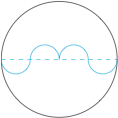# 每日一题太极函数

A．对于任意一个圆，其对应的太极函数不唯一

B．如果一个函数是两个圆的太极函数，那么这两个圆是同心圆

C．圆 $(x-1)^2+(y-1)^2=4$ 的一个太极函数是 $f(x)=x^3-3x^2+3x$

D．圆的太极函数均是中心对称图形AC．